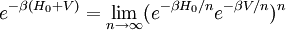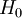# Trotter formula$e^{-\beta (H_0 + V)} = \lim_{n \rightarrow \infty} (e^{-\beta H_0 /n} e^{-\beta V/n})^n$
where$H_0$ and$V$ are self-adjoint operators. This formula is used in the path integral formulation.